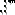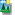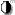# instantreality 1.0

Component:
Rendering
Status:
fully implemented
Structure type:
concrete
Standard:
X3D3.1

### IndexedTriangleFanSet

IndexedTriangleFanSet is a geometry node that can contain a Color, Coordinate, Normal and TextureCoordinate node. Hint: insert a Shape node before adding geometry or Appearance. You can also substitute a type-matched ProtoInstance for content.

#### Code

##### XML encoding
```<IndexedTriangleFanSet index=''
lit='TRUE'
normalIndex=''
colorIndex=''
texCoordIndex=''
normalPerVertex='TRUE'
colorPerVertex='TRUE'
ccw='TRUE'
creaseAngle='0'
solid='TRUE'
showNormalLength='0'
resolution='1'
multiResolutionLevel='1'
invalidateVolume='FALSE'
normalUpdateMode='nice'
cacheMode='auto'
optimizationMode='auto'
logFeature=''
/>```
##### Classic encoding
```IndexedTriangleFanSet {
index []
lit TRUE
normalIndex []
colorIndex []
texCoordIndex []
normalPerVertex TRUE
colorPerVertex TRUE
ccw TRUE
creaseAngle 0
solid TRUE
showNormalLength 0
resolution 1
multiResolutionLevel 1
invalidateVolume FALSE
normalUpdateMode "nice"
cacheMode "auto"
optimizationMode ["auto"]
logFeature [""]
}
```

#### Interface

Filter: X3D only | Avalon only | All
id Name DataType PartType Default ValueType Descriptionindex MFInt32 inputOutput index specifies triangles by connecting Coordinate vertices.normalIndex MFInt32 inputOutput Interchange profile hint: this field may be ignored.colorIndex MFInt32 inputOutput colorIndex indices provide order in which colors are applied. Hint: if rendering Coordinate points originally defined for an IndexedFaceSet, index values may need to repeat initial each initial vertex to close the polygons.texCoordIndex MFInt32 inputOutput List of texture-coordinate indices mapping attached texture to corresponding coordinates. Hint: use a tool!coord SFNode inputOutput Coordinate Coordinate node specifiying the vertices used by the geometry.normal SFNode inputOutput Normal If not NULL, it shall contain a Normal node whose normals are applied to the vertices or faces of the geometry.color SFNode inputOutput Color If NULL the geometry is rendered using the Material and texture defined in the Appearance node. If not NULL the field shall contain a Color node whose colours are applied depending on the value of "colorPerVertex".texCoord SFNode inputOutput TextureCoordinateBase If not NULL, it shall contain a TextureCoordinate node.fogCoord SFNode inputOutput FogCoordinate If not NULL, it shall contain a FogCoordinate node.attrib MFNode inputOutput VertexAttribute If the "attrib" field is not empty it shall contain a list of per-vertex attribute information for programmable shadersnormalPerVertex SFBool initializeOnly TRUE Whether Normal node is applied per vertex (true) or per polygon (false).colorPerVertex SFBool initializeOnly TRUE Whether Color node is applied per vertex (true) or per polygon (false).ccw SFBool initializeOnly TRUE ccw = counterclockwise: ordering of vertex coordinates orientation. Hint: "ccw" false can reverse solid (backface culling) and normal-vector orientation.creaseAngle SFFloat initializeOnly 0 [0, Inf) "creaseAngle" defines angle (in radians) for determining whether adjacent polygons are drawn with sharp edges or smooth shading. If angle between normals of two adjacent polygons is less than "creaseAngle", smooth shading is rendered across the shared line segment. Interchange profile hint: only 0 and radians supported. Hint: creaseAngle=0 means render all edges sharply, creaseAngle=3.14 means render all edges smoothly.solid SFBool initializeOnly TRUE Setting "solid" true means draw only one side of polygons (backface culling on), setting "solid" false means draw both sides of polygons (backface culling off). Warning: default value true can completely hide geometry if viewed from wrong side!metadata SFNode inputOutput MetadataObject container for payload metadata inside MetadataSet element